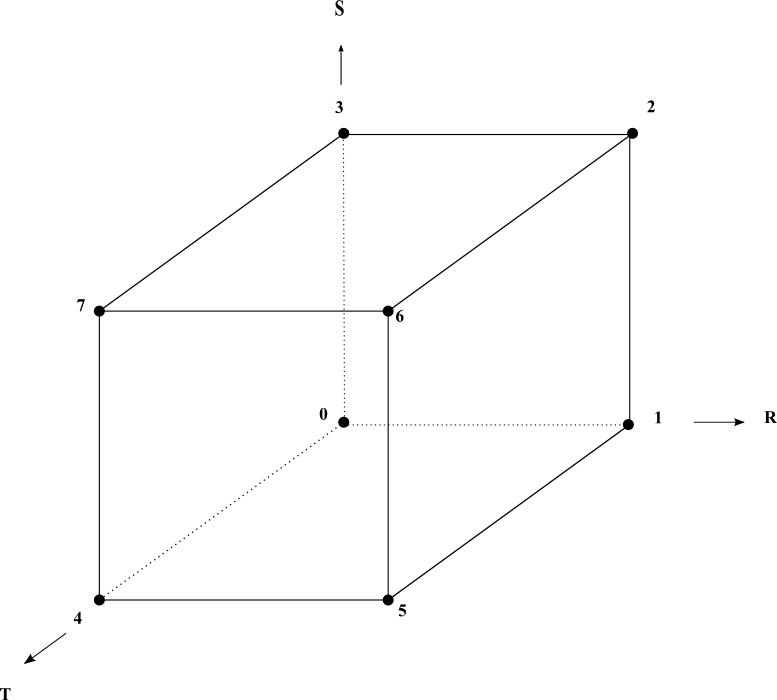## Class MxHexahedronCellExtract

• ```public class MxHexahedronCellExtract
extends MxCellExtract```
Utility class for hexahedron cells.

Utility class that provides a static implementation of `MiVolumeCell` interface methods for a hexahedron cell. This class is provided to make it easier to create a class that implements the `MiVolumeCell` interface for a hexahedron cell.

The following image shows the node numbering and the face numbering used by this class. The isosurface topology ( `getIsosurfTopology` ) is defined according to this node numbering.

Nodes and faces numbering• ### Method Summary

All Methods
Modifier and Type Method Description
`static int[]` ```getIsosurfTopology​(int caseId, int[] edges)```
Returns the isosurface topology of the associated case Id.
`static double` ```getLongestEdgeLength​(MiGeometryI meshGeometry, MiVolumeCell cell)```
Gets the longest edge of a hexahedron cell.
`static boolean` ```isPointInsideCell​(MiGeometryI meshGeometry, MiVolumeCell hexahedronCell, double[] point, double[] weights)```
Checks if a point is inside or outside an hexahedron cell.
• ### Methods inherited from class com.openinventor.meshvizxlm.extractors.cell.MxCellExtract

`getCenter`
• ### Methods inherited from class java.lang.Object

`equals, getClass, hashCode, notify, notifyAll, toString, wait, wait, wait`
• ### Method Detail

• #### isPointInsideCell

```public static boolean isPointInsideCell​(MiGeometryI meshGeometry,
MiVolumeCell hexahedronCell,
double[] point,
double[] weights)```
Checks if a point is inside or outside an hexahedron cell.

This static method helps to implement the method `MiCell.isPointInsideCell(MiGeometryI, double[], double[])` for a hexahedron cell.

Parameters:
`meshGeometry` - the geometry of the mesh
`hexahedronCell` - the input cell.
`point` - the input point given in the same space coordinate as meshGeometry
`weights` - the array into which the weight values are to be stored. This method computes the 8 values weights[0-7] if the point is inside the cell. It assumes the weights array has been already allocated. Its size must be set to 8 (at least) before calling this method
Returns:
`true` if the point is inside the cell
• #### getLongestEdgeLength

```public static double getLongestEdgeLength​(MiGeometryI meshGeometry,
MiVolumeCell cell)```
Gets the longest edge of a hexahedron cell.
Parameters:
`meshGeometry` - the geometry of the mesh
`cell` - the input cell
Returns:
the longest edge of the specified cell
• #### getIsosurfTopology

```public static int[] getIsosurfTopology​(int caseId,
int[] edges)```
Returns the isosurface topology of the associated case Id.

This method can be used in the `getIsosurfTopology` implementation of an hexahedron cell interface to return the information to the isosurface extraction module.

Parameters:
`caseId` - the isosurf "marching cube" entry for the cell
`edges` - The array into which the isosurface topology is to be stored: array of node indices ordered by pair defining an edge, polygons are separated by `MxMeshViz.END_LIST`, the end of the polygon list is indicated by a pair of `MxMeshViz.END_LIST`.
Returns:
The array into which the isosurface topology is stored.
Throws:
`java.lang.IllegalArgumentException` - if the caseId `>` 0xFF